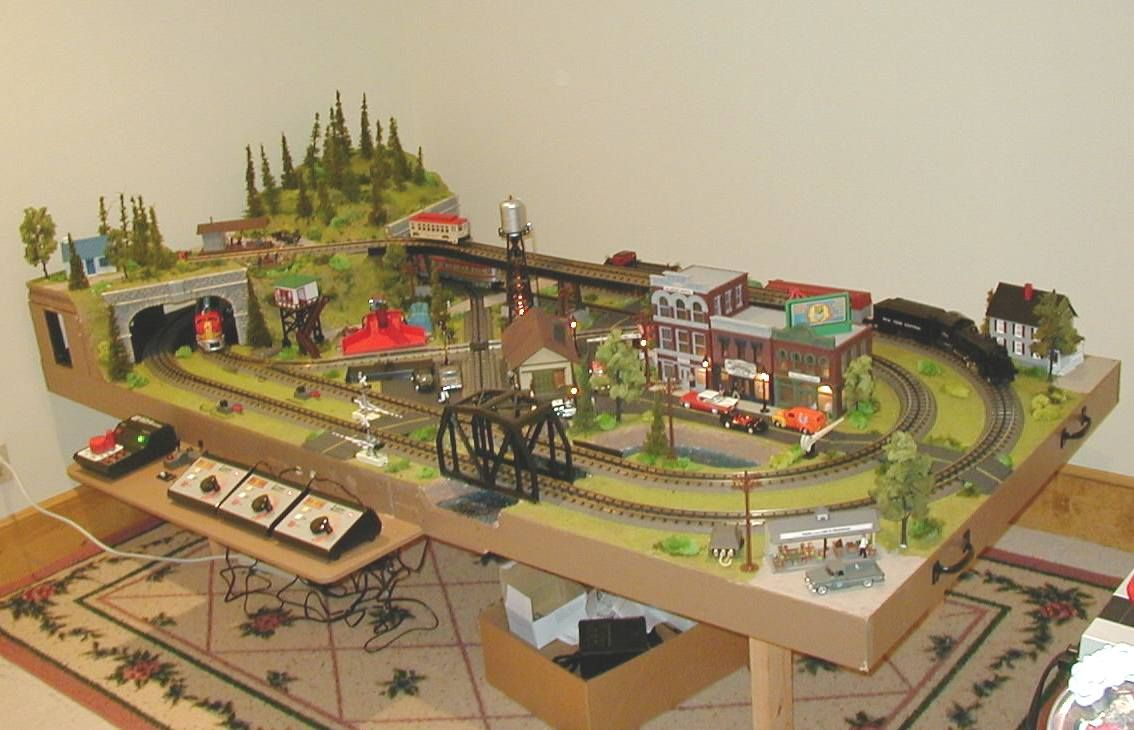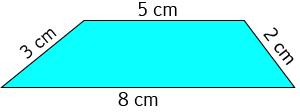# Converting LengthsSam and Jasmin have decided that they'd like to build Christo a table for his model trains as a birthday present. They've designed the table top to measure $1.7$ metres by $1.2$ metres, and are looking for someone to cut a piece of timber this size for them. The problem is that the measurements need to be in millimetres, and their measurements are in metres. So, Sam and Jasmin need to learn how to convert their measurements from metres to millimetres.

Learning to convert length measurements is a very useful skill, and one that we should all spend some time acquiring. In this article we'll look at how we can convert lengths between various units in the metric system. At the end, we'll talk about converting lengths from metric units to Imperial units and vice versa.

## Converting Metric Lengths to other Metric Units

### Centimetres to Millimetres and Back Again

Each centimetre is made up of $10$ millimetres.

To convert a measurement from centimetres to millimetres, you need to multiply it by $10$. For example, a measurement of $23$ centimetres would convert to $23 \times 10 = 230$ millimetres.

To convert a measurement from millimetres to centimetres, you need to remember that the millimetre is the smaller unit, so you need to divide by $10$. A measurement of $366$ millimetres would become $366 \div 10 = 36.6$ centimetres.

### Metres to Centimetres and Back Again

Each metre is made up of $100$ centimetres. So, to convert a length measurement from metres to centimetres, you simply need to multiply it by $100$. For example, Sam and Jasmin's table top has a length of $1.7$ metres. This is $1.7 \times 100 = 170$ centimetres.

To convert a measurement from centimetres to metres, you need to remember that each metre is made up of $100$ centimetres, so you need to divide your measurement by $100$. For example, a measurement of $280$ centimetres would become $280 \div 100 = 2.8$ metres.

### Metres to Millimetres and Back Again

We know that there are $100$ centimetres in a metre and $10$ millimetres in a centimetre. Combining these two facts together tells us that there are $100 \times 10 = 1,000$ millimetres in a metre.

To convert a measurement from metres to millimetres, simply multiply it by $1,000$. The length of Sam and Jasmin's table top is $1.7 \times 1,000 = 1,700$ millimetres. Its width is $1.2 \times 1,000 = 1,200$ millimetres.

Sometimes, we need to convert measurements in millimetres to measurements in metres. To do this, remember that millimetres are smaller than metres and divide the number of millimetres by $1,000$. For example, a measurement of $12,500$ millimetres would convert to a measurement of $12,500 \div 1,000 = 12.5$ metres.

### Metres to Kilometres and Back Again

There are $1,000$ metres in one kilometre.

To convert a distance from kilometres to metres, multiply by $1,000$. For example, a distance of $3.8$ kilometres would convert to a distance of $3.8 \times 1,000 = 3,800$ metres.

To convert a distance from metres to kilometres, we need to divide by $1,000$ (metres are smaller than kilometres). So, if someone told you that the distance from their home to their school was $2,600$ metres, you could convert this to a distance of $2,600 \div 1,000 = 2.6$ kilometres.

### Example: Doing Conversions in Geometry ProblemsHere's a problem out of Sam's maths text book that he'd like some help with solving. He's been asked to find the perimeter of the trapezium in millimetres.

Well Sam, the first thing you should do is find the perimeter in centimetres as all the measurements are given in centimetres. Simply add up the lengths of the four sides to give $3 + 5 + 2 + 8 = 18$ centimetres.

Next, multiply this perimeter by $10$ as each centimetre is made up of $10$ millimetres. The answer is simply $18 \times 10 = 180$ millimetres.

## Converting between Metric and Imperial Length Units.

We can convert between metric and Imperial lengths using similar methods. We just need to know what number to multiply each unit by to give the conversion.

For example, one foot is $0.3048$ metres. So, to convert a length of $10$ feet to metres, we simply multiply it by $0.3048$ to give $10 \text{ feet} = 10 \times 0.3048 = 3.048 \text{ metres}$.

You can convert a distance in kilometres into miles by multiplying it by $0.6214$. So, a distance of $100$ kilometres is equal to $100 \times 0.6214 = 62.14$ miles.

As a final example, one inch is the same as $25.4$ millimetres. So, a $10$ inch long piece of ribbon is a piece of ribbon that is $10 \times 25.4 = 254$ millimetres long.

You can do similar conversions between any Imperial unit of length and any metric unit of length, and vice versa. You just need to know the conversion factors (numbers to multiply by). Have fun!

### Description

• Metric numbers
• Speed
• Conversions

and several other topics

### Audience

Year 10 or higher, some chapters are suitable for Year 8+ students

### Learning Objectives

Learn about measurement and related topics

Author: Subject Coach
You must be logged in as Student to ask a Question.